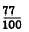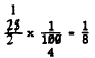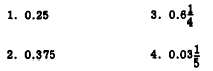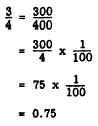Changing decimals to common fractionsCustom SearchCHANGING DECIMALS TO COMMON FRACTIONS Any decimal may be reduced to a common fraction. To do this we simply write out the numerator and denominator in full and reduce to lowest terms. For example, to change 0.12 to a common fraction, we simply write out the fraction in full,and reduce to lowest terms,Likewise, 0.77 is writtenbut this is in lowest terms 60 the fraction cannot be further reduced. One way of checking to see if a decimal fraction can be reduced to lower terms is to consider the makeup of the decimal denominator. The denominator is always 10 or a power of 10. Inspection shows that the prime factors of 10 are 5 and 2. Thus, the numerator must be divisible by 5 or 2 or both, or the fraction cannot be reduced. EXAMPLE: Change the decimal 0.0925 to a common fraction and reduce to lowest terms. SOLUTION:Complex decimals are changed to common fraction6 by first writing out the numerator and denominator in full and then reducing the resulting complex fraction in the usual way. For example, to reduce 0.12 1/2 we first WriteWriting the numerator as an improper fraction we haveand applying the reciprocal method of division, we havePractice problems. Change the following decimals to common fraction6 in lowest terms:Answers: 1. 1/4 2. 3/8 3. 5/8 4. 4/125 CHANGING COMMON FRACTIONS TO DECIMALS The only difference between a decimal fraction and a common fraction is that the decimal fraction has 1 with a certain number of zeros (in other words, a power of 10) for a denominator. Thus, a common fraction can be changed to a decimal if it can be reduced to a fraction having a power of 10 for a denominator. If the denominator of the common fraction in its lowest terms is made up Of the prime factors 2 or 5 or both, the fraction can be converted to an exact decimal. If some other prime factor is present, the fraction cannot be converted exactly. The truth of this is evident when we Consider the denominator of the new fraction. It must always be 10 or a power of 10, and we know the factor6 of such a number are always 2’s and 5’s. The method of converting a common fraction to a decimal is illustrated as follows: EXAMPLE: Convert 3/4 to a decimal. SOLUTION:Notice that the original fraction could have been rewritten as 3000/4000, in which case the result would have been 0.750. On the other hand, if the original fraction had been rewritten as 30/40, the resulting division of 4 into 30 would not have been po66ible without a remainder. When the denominator in the original fraction has only 2’s and 5's as factors, so that we know a remainder is not necessary, the fraction should be rewritten with enough 0's to complete the division with no remainder. Observation of the results In the foregoing example lead6 to a shortcut in the conversion method. Nothing that the factor l/100 ultimately enters the answer in the form of a decimal, we could introduce the decimal point as the final step without ever writing the fraction 1/100. Thus the rule for changing fraction6 to decimals is as follows: 1. Annex enough 0's to the numerator of the original fraction 60 that the division will be exact (no remainder). 2. Divide the original denominator into the new numerator formed by annexing the 0's 3. Place the decimal-point in the answer so that the number of decimal places in the answer is the same as the number of 0’s annexed to the original numerator. If a mixed number in common fraction form is to be converted, convert only the fractional part and then write the two parts together. This is illustrated as follows:Practice problems. Convert the following common fractions and mixed numbers to decimal form:Answers: 1. 0.25  2. 0.375  3. 0.15625  4. 2.3125Integrated Publishing, Inc. - A (SDVOSB) Service Disabled Veteran Owned Small Business